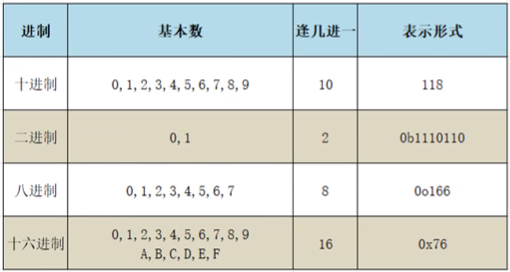• 十进制---Python默认的进制
• 二进制---以0b开头（计算机默认的进制）
• 八进制---以0o开头
• 十六进制---以0x开头print('十进制', 118)  # python默认进制，范围0-9
print('二进制', 0b10101111)  # 计算机默认进制，范围0和1
print('八进制', 0o166)  # 范围0-7
print('十六进制', 0xed76)  # 范围0-9和a-f
# 这样的输出形式都会输出为十进制，可用程序员计算机计算是否相符

• 十进制 118
• 二进制 175
• 八进制 118
• 十六进制 60790

1.逢几进一

2.借一当几

print(0b101011 + 1)  # 二进制：0b101011+1=0b101100 逢二进一
print(0b101010 - 1)  # 二进制：0b101010-1=0b101001 借一当二
print(0o167 + 1)  # 八进制：0o167+1=0o170 逢八进一
print(0o160 - 1)  # 八进制：0o160-1=0o157 借一当八
print(0xed6f + 1)  # 十六进制：0xed6f+1=0xed70 逢十六进一
print(0xed60 - 1)  # 十六进制：0xed60-1=0xed5f 借一当十六

• 44
• 41
• 120
• 111
• 60784
• 60767

print(1.1 + 2.2)  # 测试来看，计算结果小数部分有3就会出现不精确的情况
print(1.1 + 2.1)

• 3.3000000000000003
• 3.2

from decimal import Decimal  # 调用模块的表达式

a = Decimal('1.1') + Decimal('2.2')  # 这里用赋值是为了方便查看输出结果的数据类型
print(a + 1, type(a))  # 输出的数据类型虽然不是整数和浮点数类型，但允许进行加减乘除的运算

• 4.3 <class 'decimal.Decimal'>

（未理解，后期跟进）

• True = 1
• False = 0
a = True
b = False
print(a + 1)
print(b + 1)
print(float(a))
print(float(b))

• 2
• 1
• 1.0
• 0.0

print('11112222')
print('1111\n2222')
print("11112222")
print('''1111
2222''')
print("""11112222""")

• 11112222
• 1111
2222
• 11112222
• 1111
2222
• 11112222

q1 = 'ooo'
q2 = 'ppp'
print(q1 * 3 + q2)

• oooooooooppp